Скачать презентацию CS 4731 Computer Graphics Lecture 24 Color Science

bd6c6d74c2e9ef45cdb1dab787df31b6.ppt

• Количество слайдов: 28CS 4731: Computer Graphics Lecture 24: Color Science Emmanuel Agu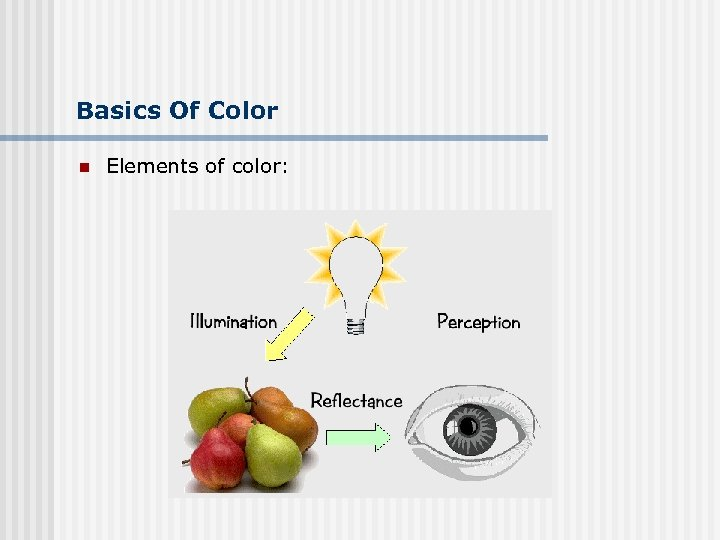Basics Of Color n Elements of color: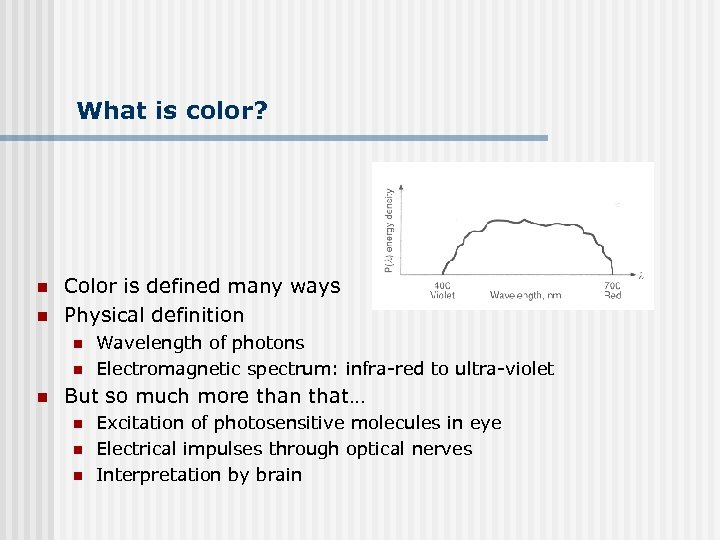What is color? n n Color is defined many ways Physical definition n Wavelength of photons Electromagnetic spectrum: infra-red to ultra-violet But so much more than that… n n n Excitation of photosensitive molecules in eye Electrical impulses through optical nerves Interpretation by brain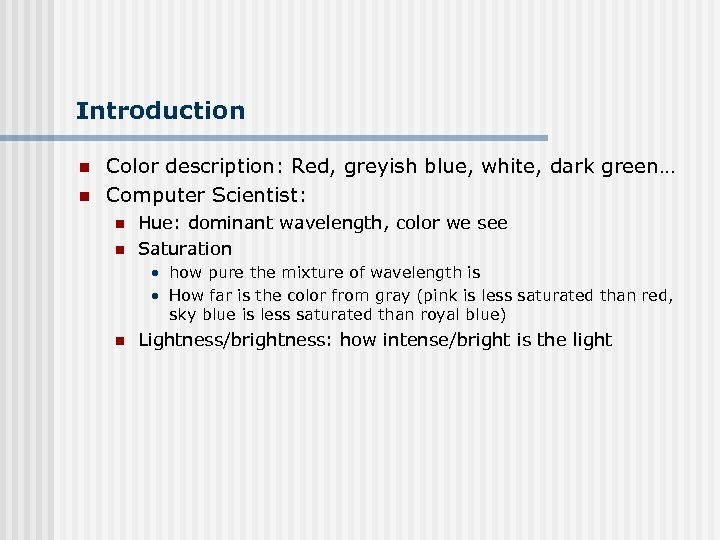Introduction n n Color description: Red, greyish blue, white, dark green… Computer Scientist: n n Hue: dominant wavelength, color we see Saturation • how pure the mixture of wavelength is • How far is the color from gray (pink is less saturated than red, sky blue is less saturated than royal blue) n Lightness/brightness: how intense/bright is the light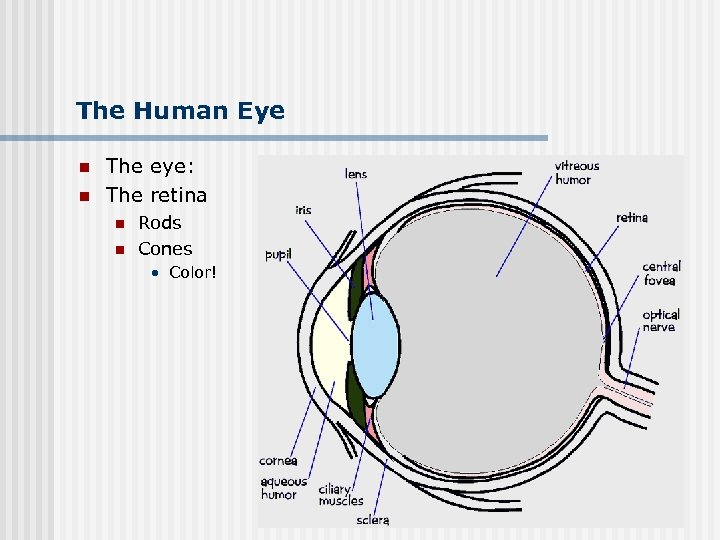The Human Eye n n The eye: The retina n n Rods Cones • Color!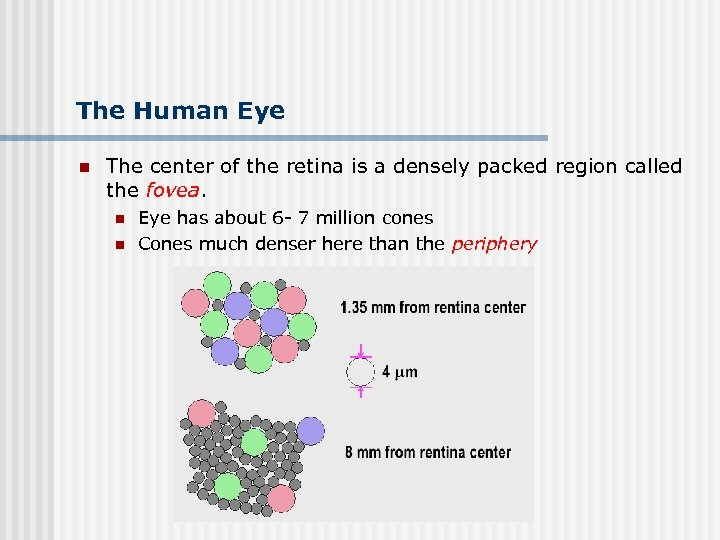The Human Eye n The center of the retina is a densely packed region called the fovea. n n Eye has about 6 - 7 million cones Cones much denser here than the periphery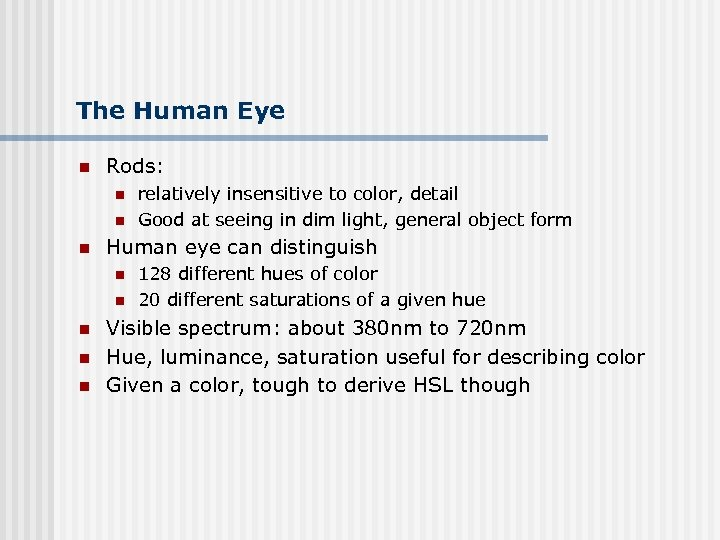The Human Eye n Rods: n n n Human eye can distinguish n n n relatively insensitive to color, detail Good at seeing in dim light, general object form 128 different hues of color 20 different saturations of a given hue Visible spectrum: about 380 nm to 720 nm Hue, luminance, saturation useful for describing color Given a color, tough to derive HSL though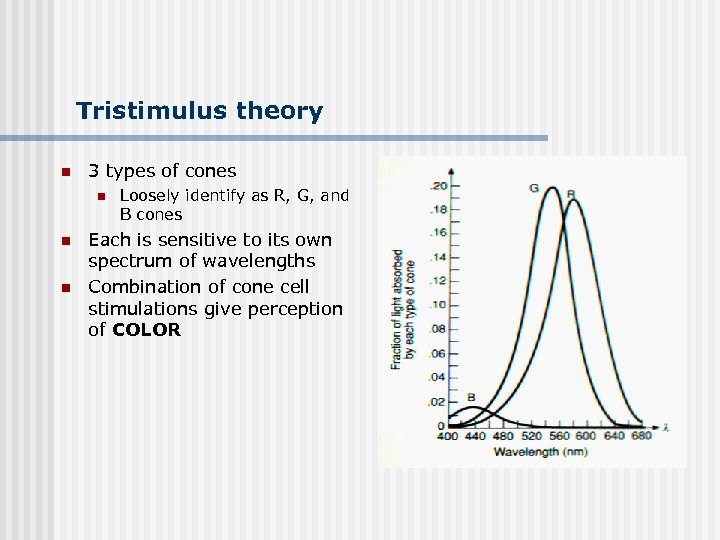Tristimulus theory n 3 types of cones n n n Loosely identify as R, G, and B cones Each is sensitive to its own spectrum of wavelengths Combination of cone cell stimulations give perception of COLOR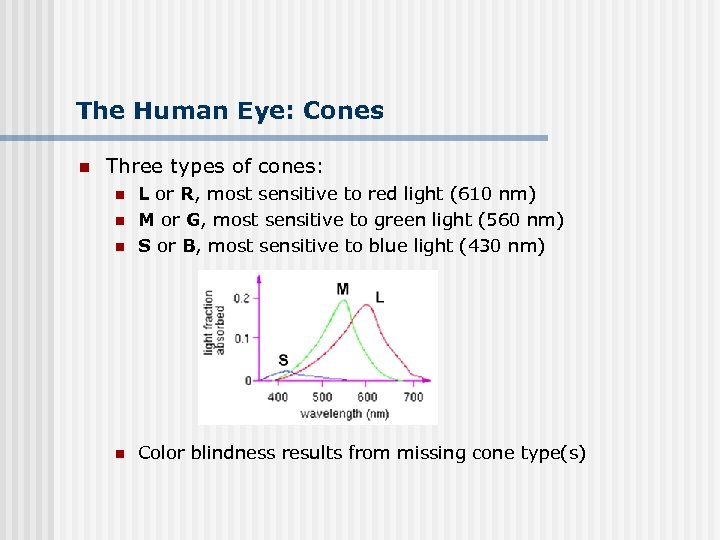The Human Eye: Cones n Three types of cones: n L or R, most sensitive to red light (610 nm) M or G, most sensitive to green light (560 nm) S or B, most sensitive to blue light (430 nm) n Color blindness results from missing cone type(s) n n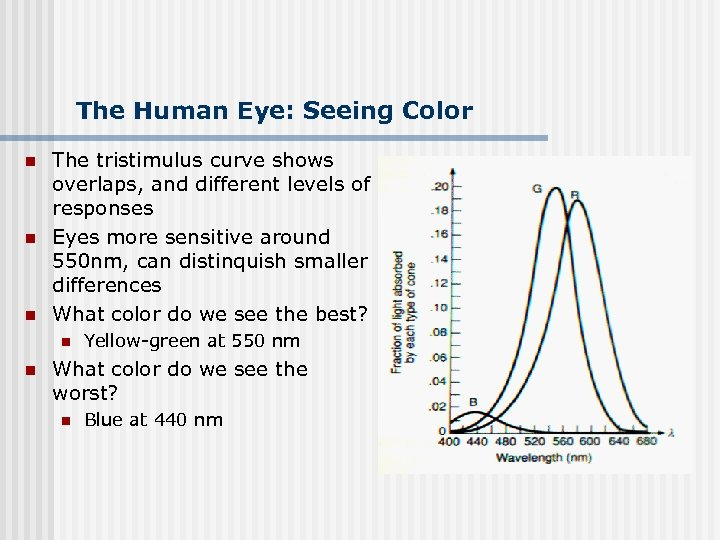The Human Eye: Seeing Color n n n The tristimulus curve shows overlaps, and different levels of responses Eyes more sensitive around 550 nm, can distinquish smaller differences What color do we see the best? n n Yellow-green at 550 nm What color do we see the worst? n Blue at 440 nm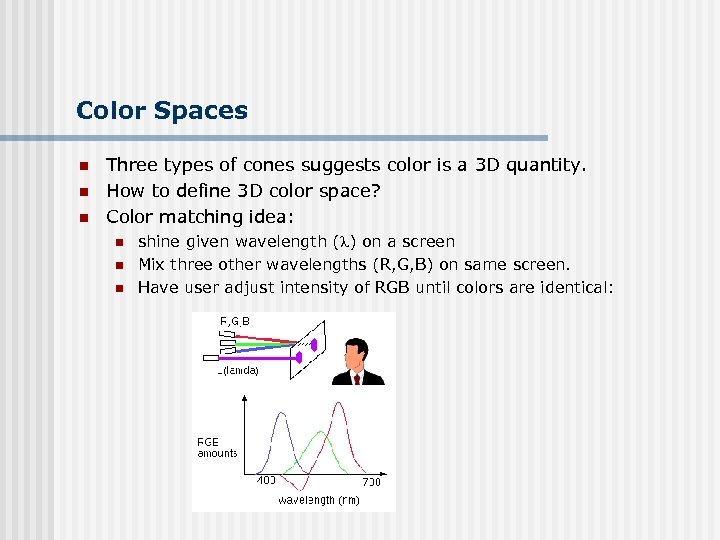Color Spaces n n n Three types of cones suggests color is a 3 D quantity. How to define 3 D color space? Color matching idea: n n n shine given wavelength ( ) on a screen Mix three other wavelengths (R, G, B) on same screen. Have user adjust intensity of RGB until colors are identical: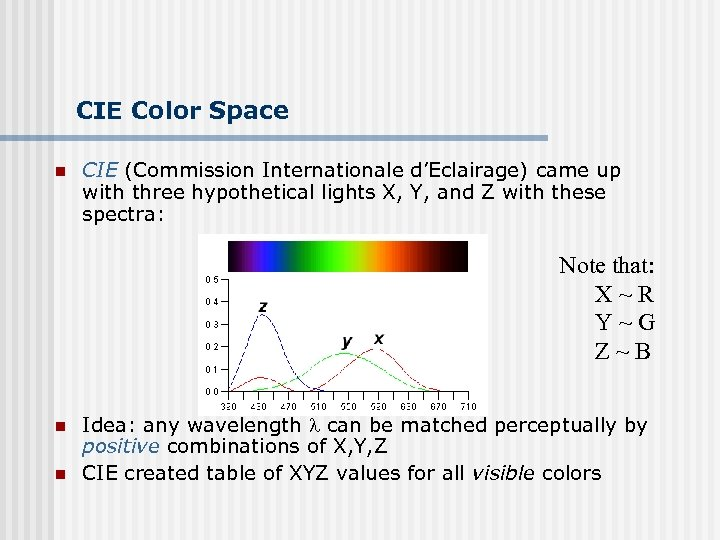CIE Color Space n CIE (Commission Internationale d’Eclairage) came up with three hypothetical lights X, Y, and Z with these spectra: Note that: X~R Y~G Z~B n n Idea: any wavelength can be matched perceptually by positive combinations of X, Y, Z CIE created table of XYZ values for all visible colors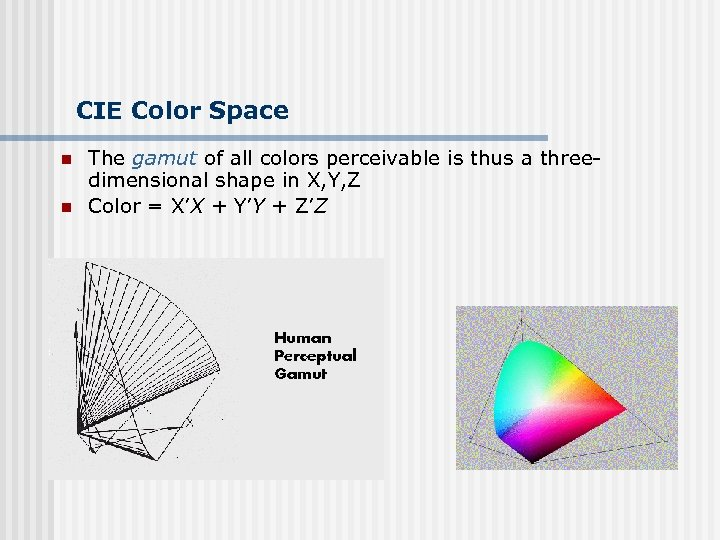CIE Color Space n n The gamut of all colors perceivable is thus a threedimensional shape in X, Y, Z Color = X’X + Y’Y + Z’Z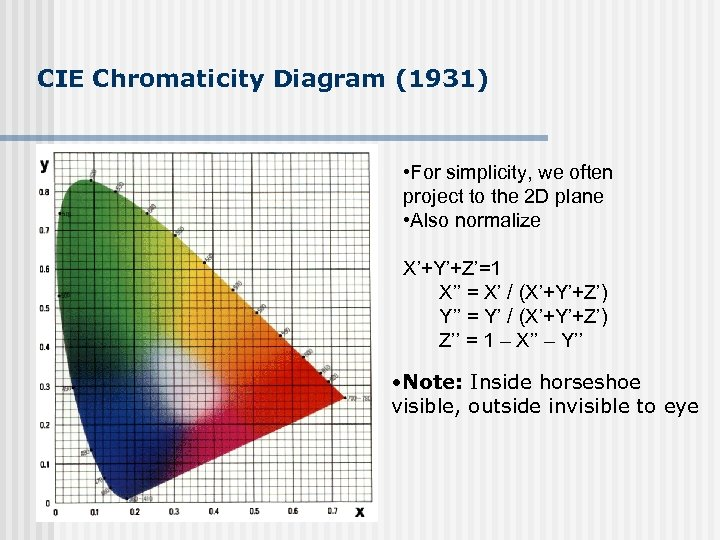CIE Chromaticity Diagram (1931) • For simplicity, we often project to the 2 D plane • Also normalize X’+Y’+Z’=1 X’’ = X’ / (X’+Y’+Z’) Y’’ = Y’ / (X’+Y’+Z’) Z’’ = 1 – X’’ – Y’’ • Note: Inside horseshoe visible, outside invisible to eye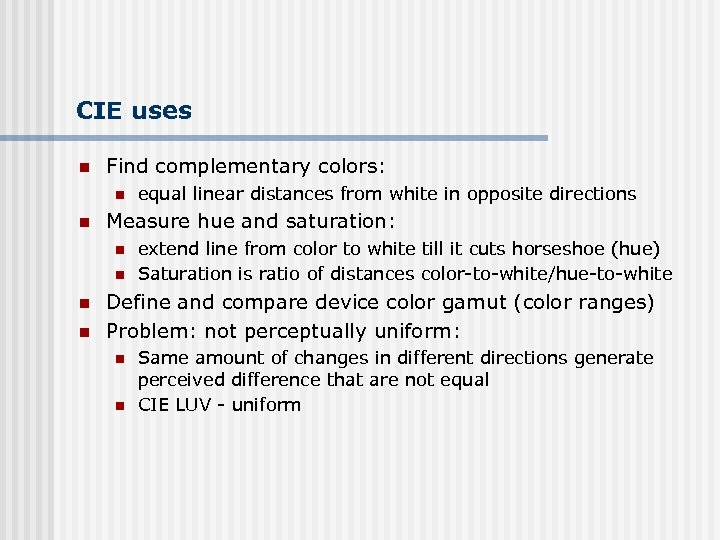CIE uses n Find complementary colors: n n Measure hue and saturation: n n equal linear distances from white in opposite directions extend line from color to white till it cuts horseshoe (hue) Saturation is ratio of distances color-to-white/hue-to-white Define and compare device color gamut (color ranges) Problem: not perceptually uniform: n n Same amount of changes in different directions generate perceived difference that are not equal CIE LUV - uniform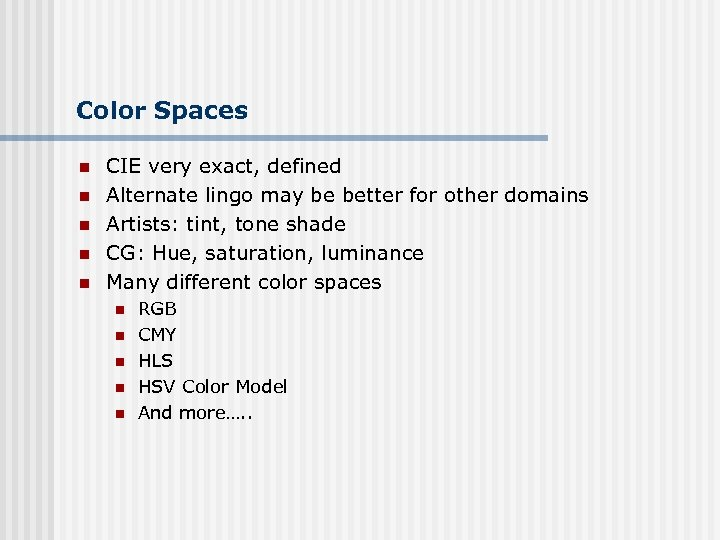Color Spaces n n n CIE very exact, defined Alternate lingo may be better for other domains Artists: tint, tone shade CG: Hue, saturation, luminance Many different color spaces n n n RGB CMY HLS HSV Color Model And more…. .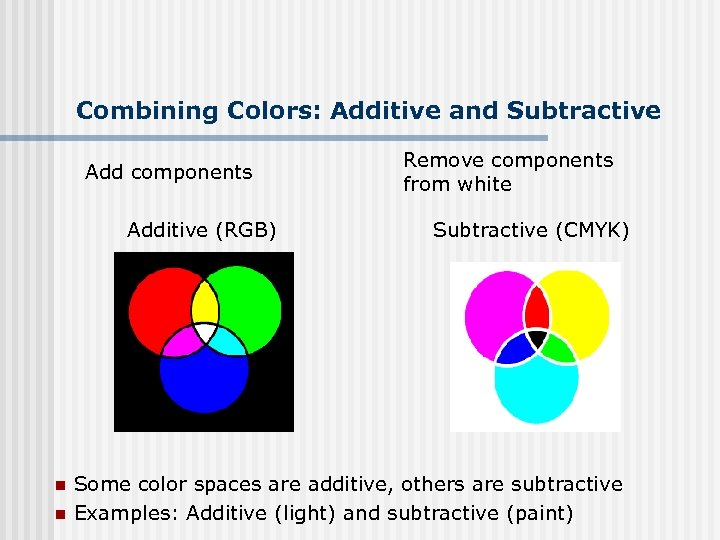Combining Colors: Additive and Subtractive Add components Additive (RGB) n n Remove components from white Subtractive (CMYK) Some color spaces are additive, others are subtractive Examples: Additive (light) and subtractive (paint)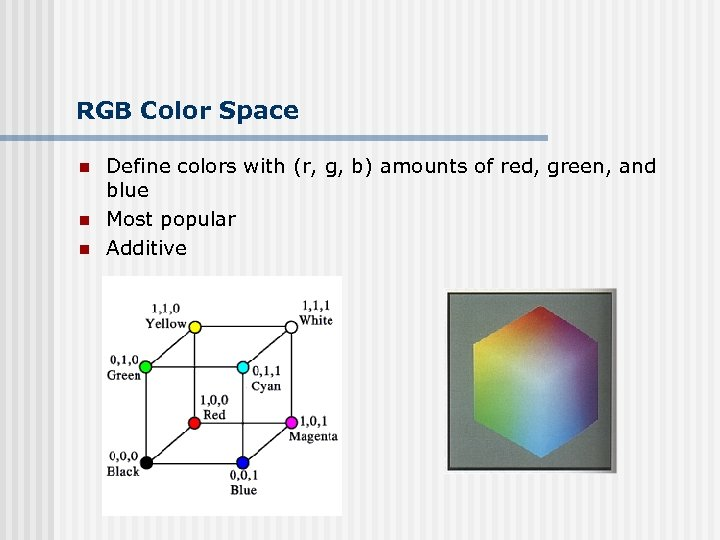RGB Color Space n n n Define colors with (r, g, b) amounts of red, green, and blue Most popular Additive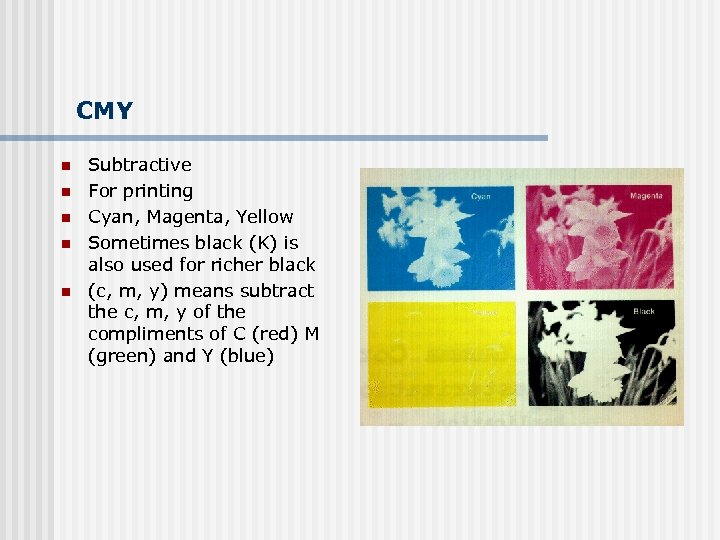CMY n n n Subtractive For printing Cyan, Magenta, Yellow Sometimes black (K) is also used for richer black (c, m, y) means subtract the c, m, y of the compliments of C (red) M (green) and Y (blue)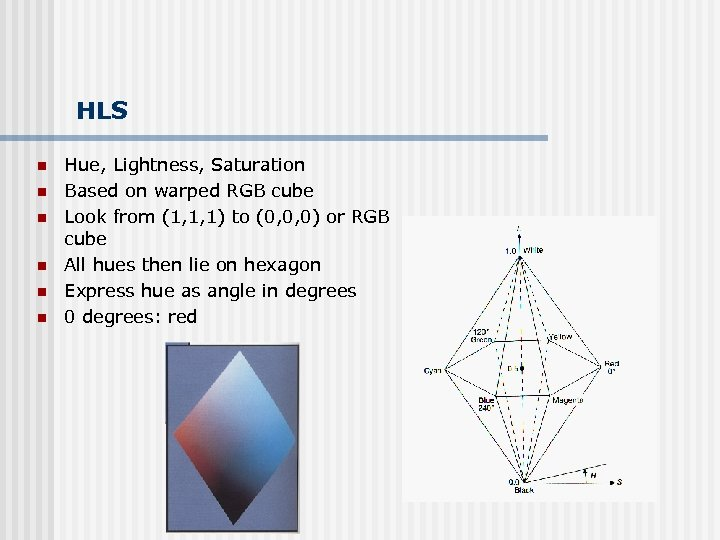HLS n n n Hue, Lightness, Saturation Based on warped RGB cube Look from (1, 1, 1) to (0, 0, 0) or RGB cube All hues then lie on hexagon Express hue as angle in degrees 0 degrees: red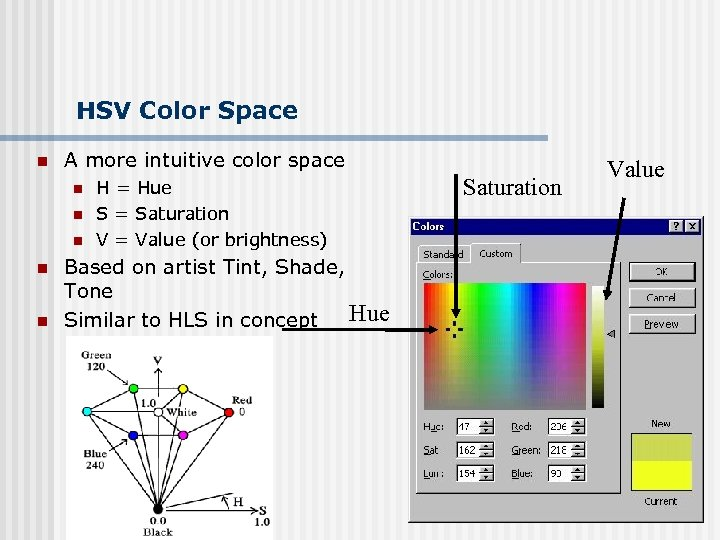HSV Color Space n A more intuitive color space n n n H = Hue S = Saturation V = Value (or brightness) Based on artist Tint, Shade, Tone Hue Similar to HLS in concept Saturation Value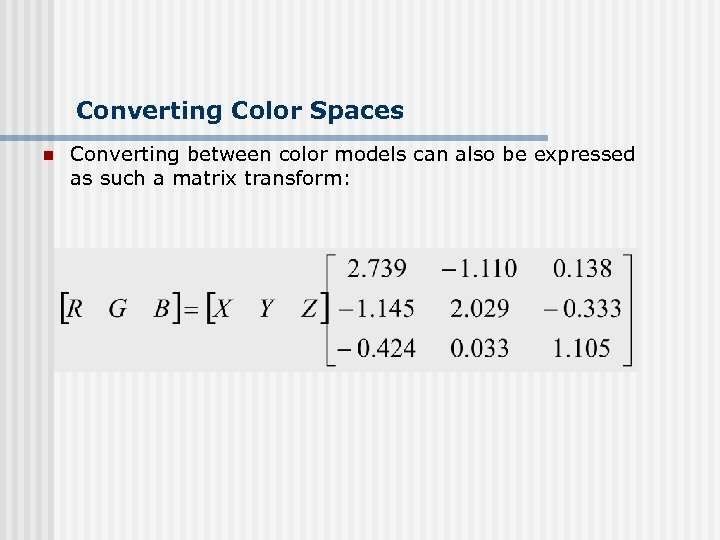Converting Color Spaces n Converting between color models can also be expressed as such a matrix transform:Color Quantization n n True color can be quite large in actual description Sometimes need to reduce size Example: take a true-color description from database and convert to web image format Replace true-color with “best match” from smaller subset Quantization algorithms: n n Uniform quantization Popularity algorithm Median-cut algorithm Octree algorithm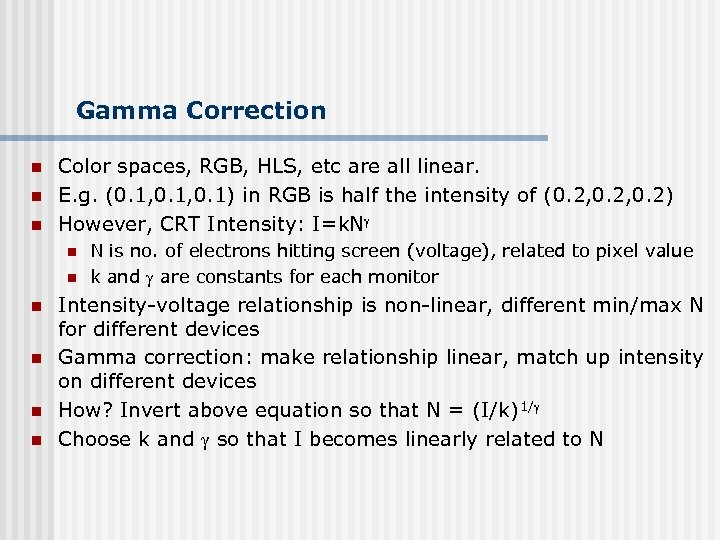Gamma Correction n Color spaces, RGB, HLS, etc are all linear. E. g. (0. 1, 0. 1) in RGB is half the intensity of (0. 2, 0. 2) However, CRT Intensity: I=k. N n n n N is no. of electrons hitting screen (voltage), related to pixel value k and are constants for each monitor Intensity-voltage relationship is non-linear, different min/max N for different devices Gamma correction: make relationship linear, match up intensity on different devices How? Invert above equation so that N = (I/k)1/ Choose k and so that I becomes linearly related to N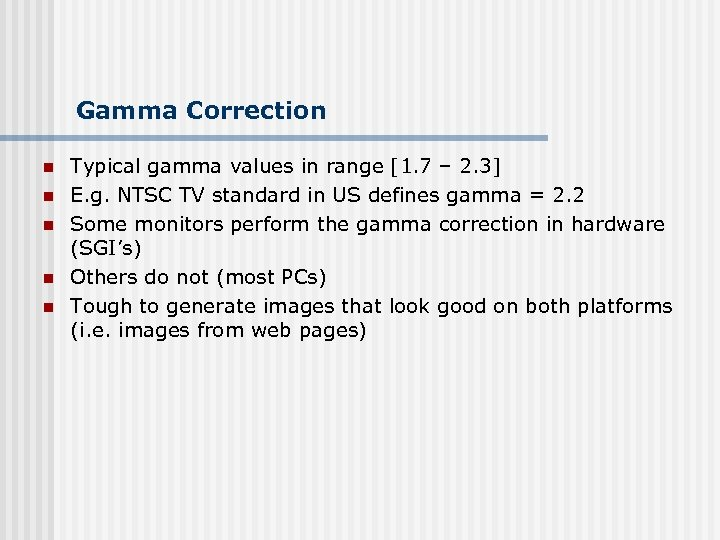Gamma Correction n n Typical gamma values in range [1. 7 – 2. 3] E. g. NTSC TV standard in US defines gamma = 2. 2 Some monitors perform the gamma correction in hardware (SGI’s) Others do not (most PCs) Tough to generate images that look good on both platforms (i. e. images from web pages)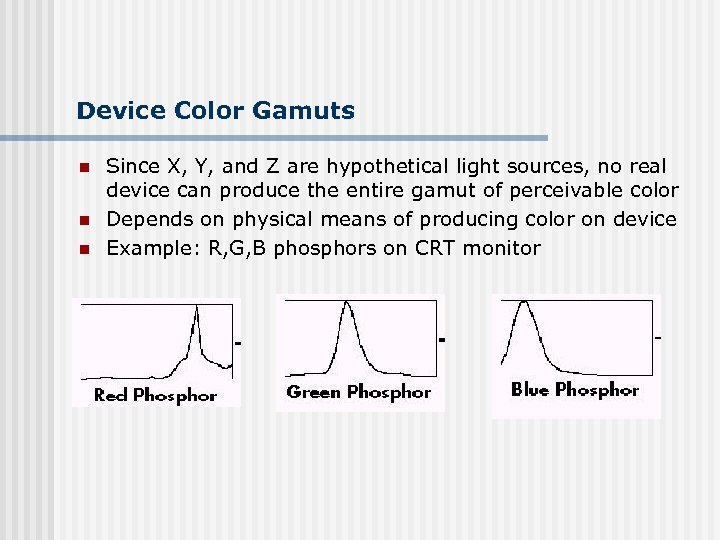Device Color Gamuts n n n Since X, Y, and Z are hypothetical light sources, no real device can produce the entire gamut of perceivable color Depends on physical means of producing color on device Example: R, G, B phosphors on CRT monitor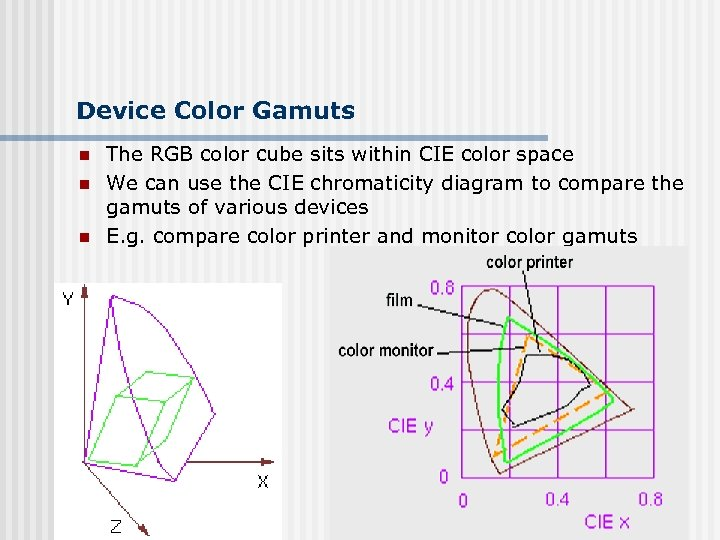Device Color Gamuts n n n The RGB color cube sits within CIE color space We can use the CIE chromaticity diagram to compare the gamuts of various devices E. g. compare color printer and monitor color gamutsReferences n Hill, chapter 12